# Binary operation of Python OpenCV image re learning and image smoothing (convolution processing)

2022-01-30 06:48:42

This article has participated in  「 Digging force Star Program 」 , Win a creative gift bag , Challenge creation incentive fund .

Python OpenCV 365 Day study plan , Go into the field of image with the eraser . This blog is the third in this series 44 piece .

## Basic knowledge

Today, let's review the last blog about binary operation , The content is still immature , Sure enough, the first time of learning just mastered the lost fur , There are still many details to be added .

### Binary learning iteration

First of all, yes `cv2.theshold` Function learning , Function prototype and parameter basis , Just read the last blog , The following contents shall be supplemented . Let's refer to the function prototype first ：

``````retval, dst = cv2.threshold(src, thresh, maxval, type[, dst])
Copy code ``````

The key parameters to be explained are `type`, Let's first review the basic usage code of basic functions , After all, it's easy to review the content with code ：

``````import cv2 as cv
import numpy as np
gray = cv.cvtColor(src, cv.COLOR_BGR2GRAY)

retval, dst = cv.threshold(gray, 127, 255, cv.THRESH_BINARY)

image = np.hstack((gray, dst))
cv.imshow("image", image)
cv.waitKey()
Copy code ``````

The operation results are as follows ：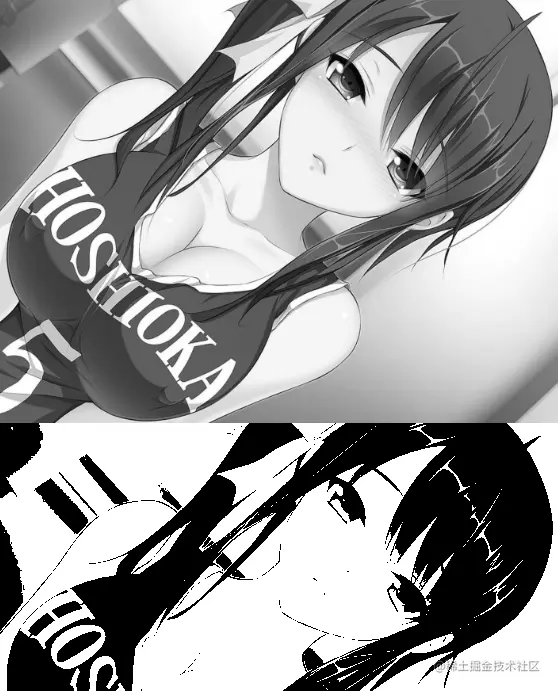the last one `type` Parameters are the key knowledge reviewed , Its value affects the result of the final binary graph . In have a look at `type` Common values are as follows 5 individual , this 5 One can also be divided into three groups , Namely

1. `THRESH_BINARY` And `THRESH_BINARY_INV`;
2. `THRESH_TRUNC`;
3. `THRESH_TOZERO` And `THRESH_TOZERO_INV`.

The two values in the first group have the same meaning as the two values in the third group , It's the opposite relationship , So just look at a parameter value .

`THRESH_BINARY` Most commonly used , Indicates that when the value of the pixel is greater than the threshold `thresh` Just take `maxval` Set the color , Generally will `thresh` Set to 127, take `maxval` Set to 255, that `THRESH_BINARY` All gray values will be greater than 127 All of them are set to 255. Note here that the binarization operation is a gray image , Although transmitting color images also works , But when doing binarization , Be sure to convert the color image into binary image in advance .

`THRESH_TOZERO` Pixels that exceed the threshold have no change , Pixels no larger than are set to 0, In fact, you can simply try .

``````import cv2 as cv
import numpy as np

cv.namedWindow("image",cv.WINDOW_FREERATIO)

gray = cv.cvtColor(src, cv.COLOR_BGR2GRAY)

retval1, dst1 = cv.threshold(gray, 127, 255, cv.THRESH_BINARY)
retval2, dst2 = cv.threshold(gray, 127, 255, cv.THRESH_TOZERO)
image = np.hstack((dst1,dst2))
cv.imshow("image", image)
cv.waitKey()
Copy code ``````

Use this method , Obviously, you can see that the gray scale in some places is worth preserving , Be careful `THRESH_TOZERO` There is no change in the pixels exceeding the threshold , No more than is set to 0 . And `THRESH_BINARY` The conclusion after comparison is , Black places are black together , Where it's white, you're whiter . So it's using `THRESH_TOZERO` When , There's no problem writing it like this .

``````retval2, dst2 = cv.threshold(gray, 127, 0, cv.THRESH_TOZERO)
Copy code ``````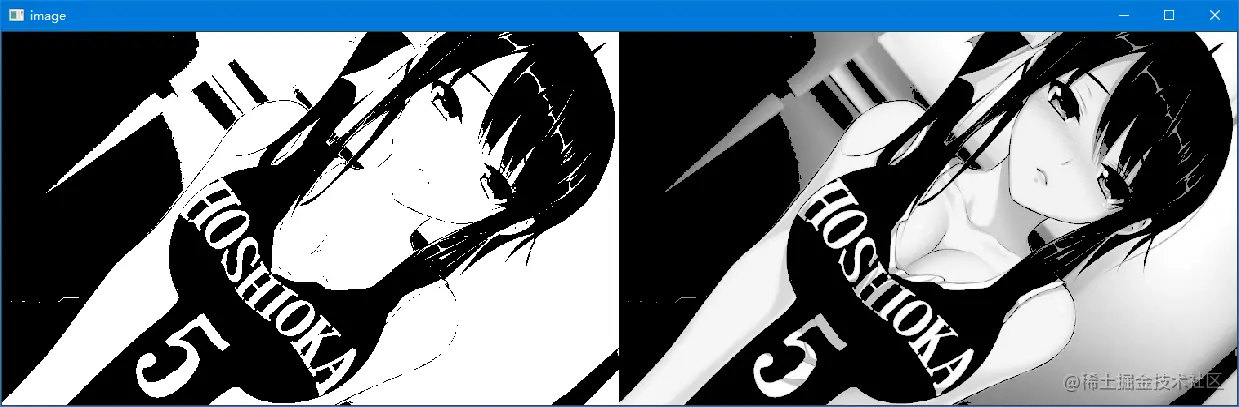`THRESH_TRUNC` Truncation thresholding , The part greater than the threshold value is set as the threshold value , Otherwise unchanged , The test code is as follows ：

``````retval1, dst1 = cv.threshold(gray, 127, 255, cv.THRESH_BINARY)
retval2, dst2 = cv.threshold(gray, 127, 0, cv.THRESH_TOZERO)
retval3, dst3 = cv.threshold(gray, 127, 0, cv.THRESH_TRUNC)
image = np.hstack((dst1,dst2,dst3))
Copy code ``````

After comparison , You can understand immediately ,`THRESH_TRUNC` The upper limit of the gray value of the picture will be set to a specific value , For example, in this case 127.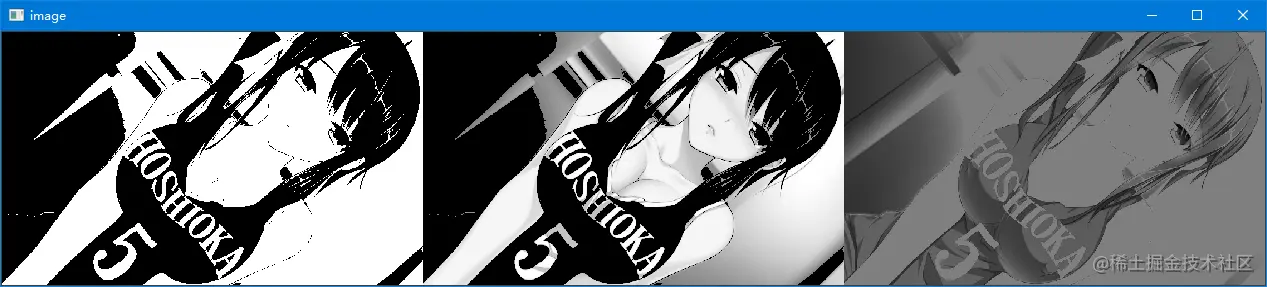### Image smoothing learning iteration

Smoothing is the convolution operation , stay This blog Both before and after , When you study again , Let's add it .

When denoising the image , Mean filtering can be used , It's simple Average convolution operation , Related to convolution Mathematics , Put... A little later 10 A few days , In addition , Because there will be convolution in many places in the next content , After learning more about the application layer , Then go to the fundamentals of Mathematics , It's twice as good as half the effort .

Although it does not involve mathematical principles , But we still need to have a basic understanding of the underlying implementation , To facilitate the realization of , I use a manually generated grayscale image to demonstrate .

Code for generating a gray image ：

``````import cv2 as cv
import numpy as np
#  Generate a 10*10 Gray scale image of
src = np.random.randint(256,size=(10, 10),dtype=np.uint8)
print(src)

cv.imshow("src",src)
Copy code ``````

Because it's randomly generated , The output code is as follows ：

``````[[ 90 134 192 243 116   2 172 143  22 218]
[192 145 171 125 175 138  64 232  90 160]
[ 61  20 231  37  77  27 141 182  71 194]
[136  86  10 239 196 137 192 243  47  40]
[220 167   3  50 227  70 135 227 225 218]
[207  10 213 134 249 157 179 112  58  78]
[107  33  68 143 124 215 175 167 108 195]
[ 32 227  43 249  61 168 230 180  82  47]
[ 89 211 253 141 199 140  34 185 179  32]
[ 18  98 109  92  37  13 200 102  97 218]]
Copy code ``````

The mean filter will select one by default `3x3` Convolution kernel , Then proceed from left to right , Convolution operation from top to bottom .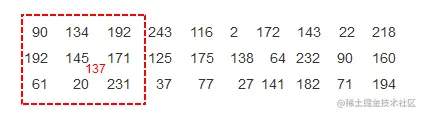The red one above 137, Is the result of mean filtering , Computing center number 145 The surrounding 9 Sum of numbers , Then divide by 9, obtain 137, Replace 145 . But you will also find a problem with this operation , That is, the edge can't get to the convolution kernel `3x3` Of , Here the eraser also consulted the relevant information , The explanation of existence is edge filling , That's what I learned in the previous blogs , But after I tried , The results are not ideal , Take time to check OpenCV Source code , Check what the calculation method is , But the core idea has been relatively clear , The mean is to calculate the average .

Because it is the convolution kernel, the average of nine values is taken to replace the middle pixel value , So the final effect is smooth , Apply it to specific images , The effect is as follows .

``````import cv2 as cv
import numpy as np

cv.namedWindow("image",cv.WINDOW_FREERATIO)

gray = cv.cvtColor(src, cv.COLOR_BGR2GRAY)

dst = cv.blur(gray,(3,3))
image = np.hstack((gray,dst))
cv.imshow("image", image)
cv.waitKey()
Copy code ``````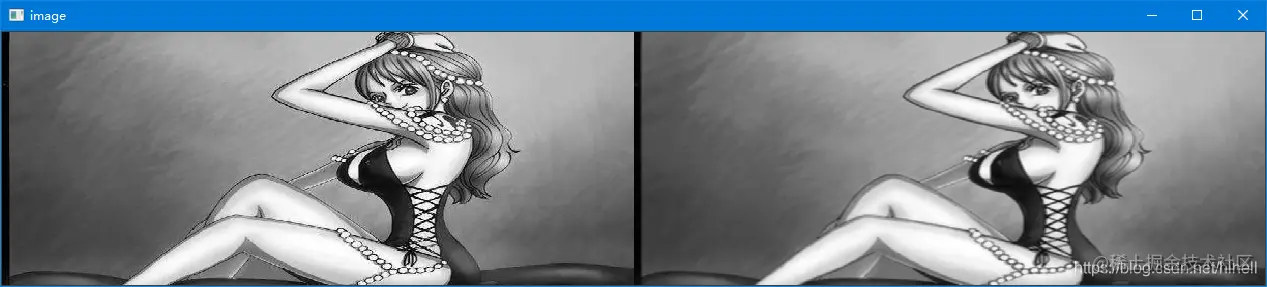The convolution kernel can be set to any size , It's just recommended to set it to `3x3``5x5` These odd numbers .

### Box filtering

In the previous blog, it seems that there is no content related to box filtering , Here is a supplement , It is basically consistent with the use of mean filtering , The function prototype is as follows ：

``````dst = cv2.boxFilter(src, ddepth, ksize[, dst[, anchor[, normalize[, borderType]]]])
Copy code ``````

You can choose whether to normalize , The specific code can run the following ：

``````#  normalization
dst = cv.boxFilter(gray,-1,(3,3),normalize=True)
#  Don't do it
dst = cv.boxFilter(gray,-1,(3,3),normalize=False)
Copy code ``````

In short , Do not normalize , In the use of `3x3` After the convolution kernel is calculated , Not divided by 9, Pixel out of bounds , By default, it is reserved as 255, It's clear .

Gaussian filtering is to increase the knowledge related to Gaussian distribution , Or added the concept of spatial distance , To put it bluntly, the pixel weight around the pixel is different . Compared to mean filtering , Gaussian filtering has better smoothing effect .

Median filtering is to sort the matrix covered by convolution kernel from small to large , Then take the median value as the pixel value of the target image .

## Eraser bars

I hope today's 1 You get something in an hour , I'll see you on our next blog ~